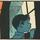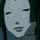## Social Question# Do you know how to calculate a weighted average?

Asked by LostInParadise (27413) July 15th, 2019

I was never taught how to do a weighted average. It is a very basic mathematical concept, which many teachers use to calculate a student’s grade.

A student gets a 95 on the final exam for a course and an 85 on the midterm. If the final counts for 60% of the grade and the midterm accounts for the remaining 40%, what is the overall score for the student? The numbers were chosen to simplify the calculation. Do you see a really simple way of doing the calculation in your head?

Observing members: 0Composing members: 0Your scenario is easy to do in one’s head, because it is a simple average weighting of only two scores. The difference between the two scores is 10 points; weighting that difference would mean the lower score plus 60% of the difference, or 6 points added to the 85 score.

But a true weighting over time of multiple scores would be more difficult to do in one’s head.

Suppose, over a course, you weighted scores as follows:

Three quizzes count for 15% each. A mid term counts for 20%. The final counts for 35%.

It is still easy to calculate the average, but takes a bit more than doing it in one’s head.

zenvelo (35042)“Great Answer” (1) Flag as…(95*.6) + (85*.4) = 57+ 34 = 91

ARE_you_kidding_me (19763)“Great Answer” (2) Flag as…I am glad to see that at least two people here are familiar with weighted averages.

Here is a problem of a type you were likely given. Do you see how it relates to weighted average? If a car travels for 3 hours at 60 mph and 2 hours at 50 mph, what was its average speed for the whole trip?

Granted it is not a very exciting problem, but seeing how it relates to weighted average should make it at least a little more interesting.

LostInParadise (27413)“Great Answer” (0) Flag as…